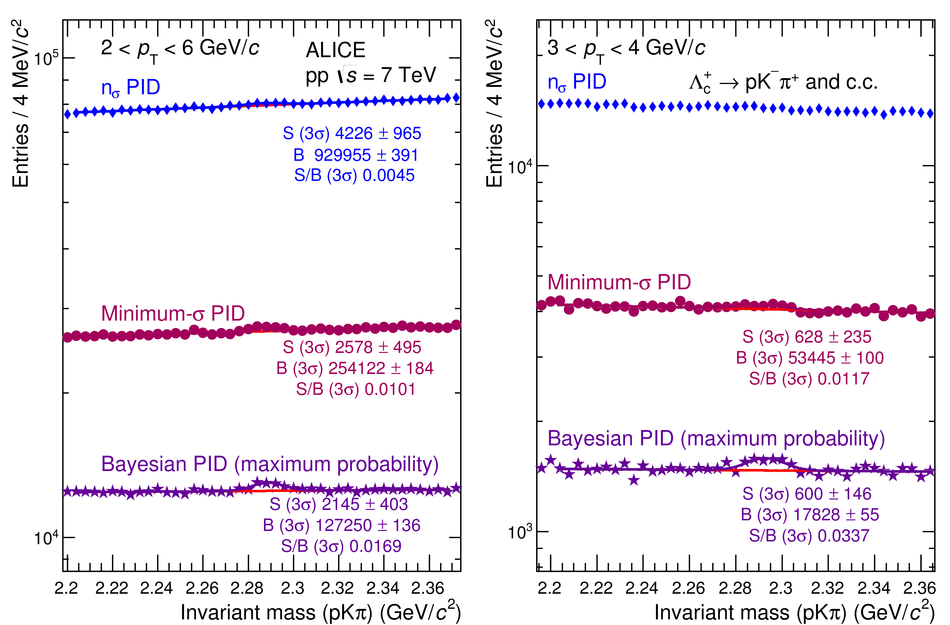# Particle identification in ALICE: a Bayesian approach

We present a Bayesian approach to particle identification (PID) within the ALICE experiment. The aim is to more effectively combine the particle identification capabilities of its various detectors. After a brief explanation of the adopted methodology and formalism, the performance of the Bayesian PID approach for charged pions, kaons and protons in the central barrel of ALICE is studied. PID is performed via measurements of specific energy loss ($\mathrm{d}E/\mathrm{d}x$) and time-of-flight. PID efficiencies and misidentification probabilities are extracted and compared with Monte Carlo simulations using high-purity samples of identified particles in the decay channels ${\rm K}^0_S \rightarrow \pi^-\pi^+$, $\phi \rightarrow {\rm K}^-{\rm K}^+$, and $\Lambda \rightarrow {\rm p}\pi^-$ in p-Pb collisions at $\sqrt{s_{\rm NN}}=5.02$ TeV. In order to thoroughly assess the validity of the Bayesian approach, this methodology was used to obtain corrected $p_{\rm T}$ spectra of pions, kaons, protons, and D$^0$ mesons in pp collisions at $\sqrt{s}=7$ TeV. In all cases, the results using Bayesian PID were found to be consistent with previous measurements performed by ALICE using a standard PID approach. For the measurement of D$^0 \rightarrow {\rm K}^-\pi^+$, it was found that a Bayesian PID approach gave a higher signal-to-background ratio and a similar or larger statistical significance when compared with standard PID selections, despite a reduced identification efficiency. Finally, we present an exploratory study of the measurement of $\Lambda_{\rm c}^{+}\rightarrow {\rm p} {\rm K}^-\pi^+$ in pp collisions at $\sqrt{s}=7$ TeV, using the Bayesian approach for the identification of its decay products.

Figures

## Figure 1

 Combined particle identification in the TPC and TOF for data from Pb-Pb collisions at $\snn$ = 2.76 TeV, shown as a two-dimensional plot. The PID signals are expressed in terms of the deviation from the expected response for pions in each detector.## Figure 2

 An example of the iterative prior extraction procedure for p-Pb data (for the 10-20$\%$ V0A multiplicity class). The extracted K$/\pi$ ratio of the priors is shown as a function of $p_{\rm T}$ at each step of the iteration (left) and as a ratio of the value between each successive step (right). Step 0 refers to the initial ratio, which is set to 1.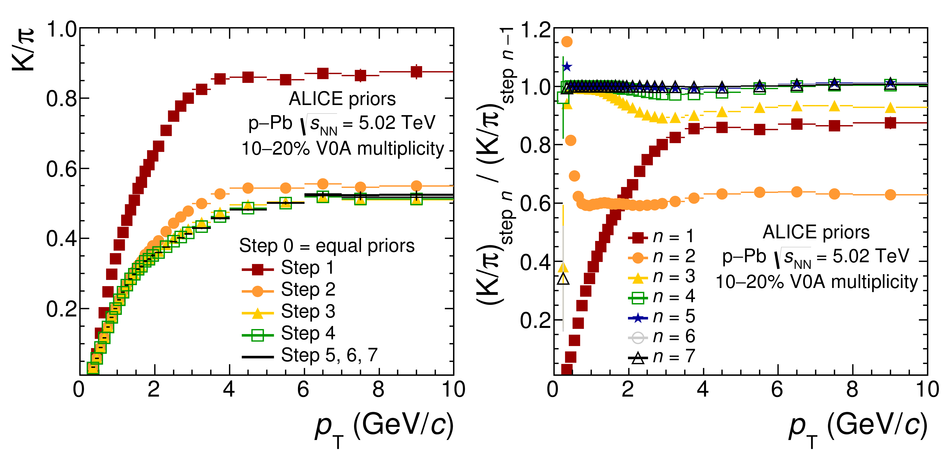## Figure 3

 The proton/pion ratio (left) and kaon/pion ratio (right), as measured by ALICE using TPC and TOF (filled symbols), compared with the standard priors as described in the text (open symbols) for Pb-Pb and p-p collisions. For Pb-Pb, the results are reported for different centrality classes. Particle ratios are calculated for mid-rapidity, $|y|< 0.5$. The double ratios (the measured abundances divided by the Bayesian priors) are shown in the lower panels.## Figure 4

 The proton/pion ratio (left) and kaon/pion ratio (right), as measured by ALICE using TPC and TOF (filled symbols),compared with the standard priors obtained with an iterative procedure (open symbols) for p-Pb collisions for different V0A multiplicity classes. Particle ratios are calculated for mid-rapidity, $|y|< 0.5$ with respect to the centre-of-mass system. The double ratios (the measured abundances divided by the Bayesian priors) are shown in the lower panels.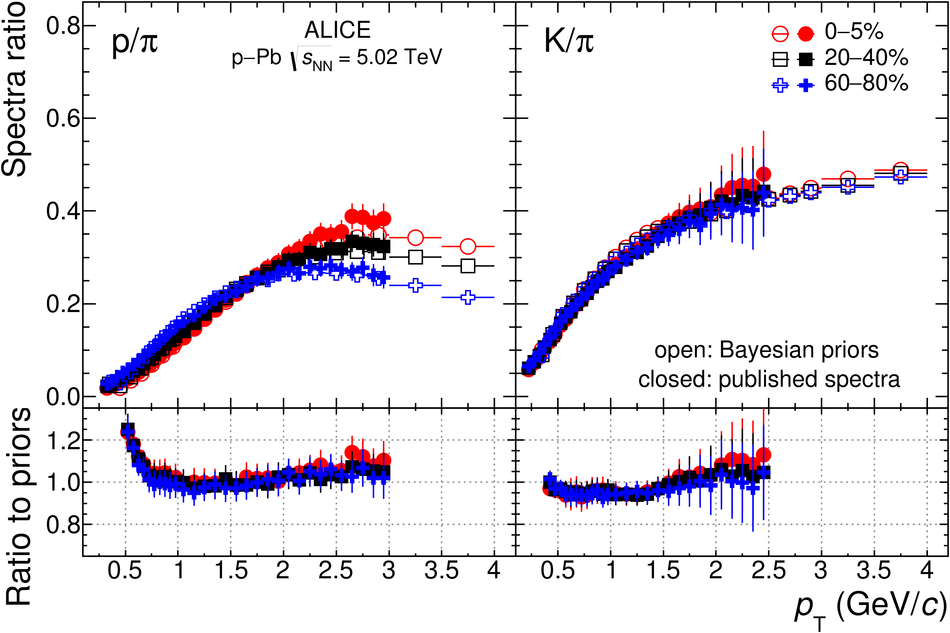## Figure 5

 V$^0$ fits to extract the yield and background for K$^{0}_{S}$ $\to$ $\pi^{-}\pi^{+}$ in p-Pb collisions at $\snn$ = 5.02 TeV. From left to right: no PID selections applied and selecting pions, kaons and protons using a specific PID strategy (here, Bayesian probability $>$ 0.2). The yield estimated from the second plot from the left (compared with the no-PID yield result) gives a measure of the PID efficiency for the pions, while the remaining ones give information about misidentification.## Figure 6

 $\varepsilon_{\rm {PID}}$ matrix elements in p-Pb collisions after selection with a Bayesian probability greater than 80$\%$. Comparisons with Monte Carlo (open symbols) are also shown.## Figure 7

 $\varepsilon_{\rm {PID}}$ matrix elements in p-Pb collisions after selection with a 2$\sigma$ selection on the combined TPC and TOF signal Comparisons with Monte Carlo (open symbols) are also shown.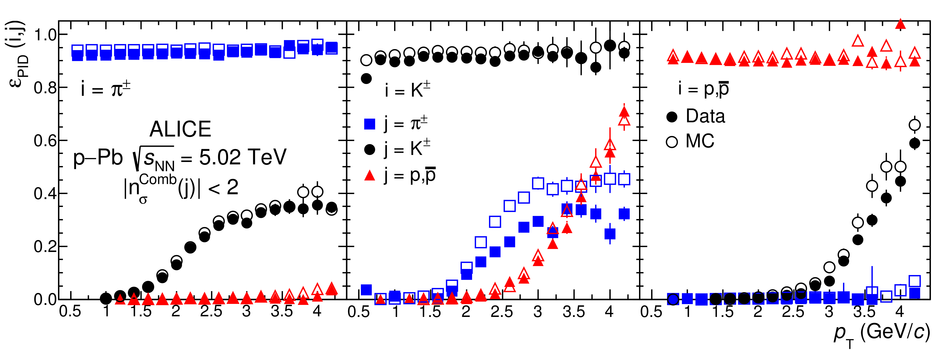## Figure 8

 Data/Monte Carlo ratios of PID efficiencies for pions, kaons and protons in p-Pb collisions, extracted using different Bayesian probability thresholds.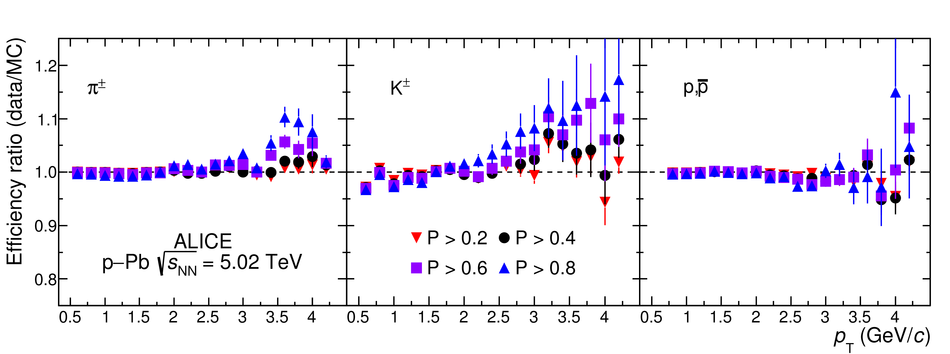## Figure 9

 Data/Monte Carlo ratios of PID efficiencies for pions, kaons and protons in p-Pb collisions, extracted using 2- and 3$\sigma$ selections on the combined TPC and TOF signal.## Figure 10

 Identified particle spectra from the Bayesian analysis, compared with the measurement reported by ALICE in pp collisions at 7 TeV.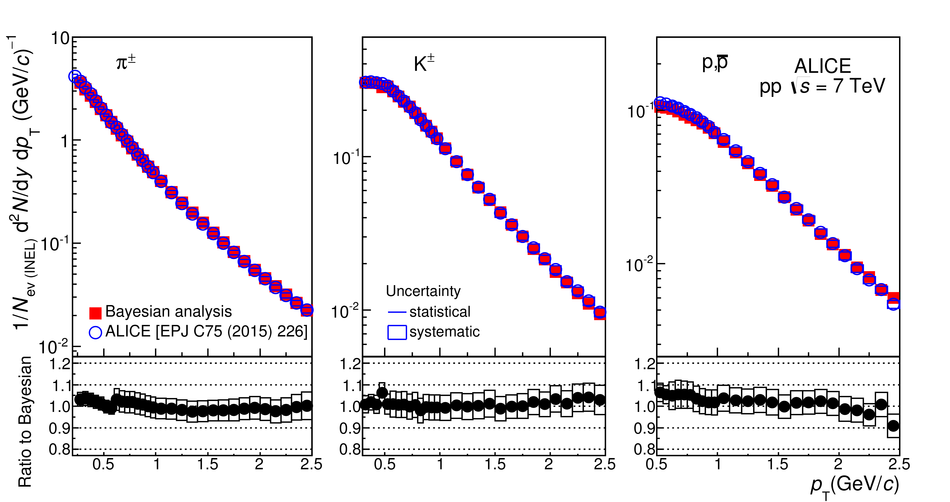## Figure 11

 A comparison of the invariant mass distributions in three $\pt$ intervals for $\Dzero$ candidates obtained without PID, with n$_{\sigma}$ PID, and with Bayesian PID using the maximum probability condition. Due to the low statistical significance, it was not possible to extract a stable signal without PID for $1< \pt< 2$ GeV/$c$, therefore this fit and its results are not shown.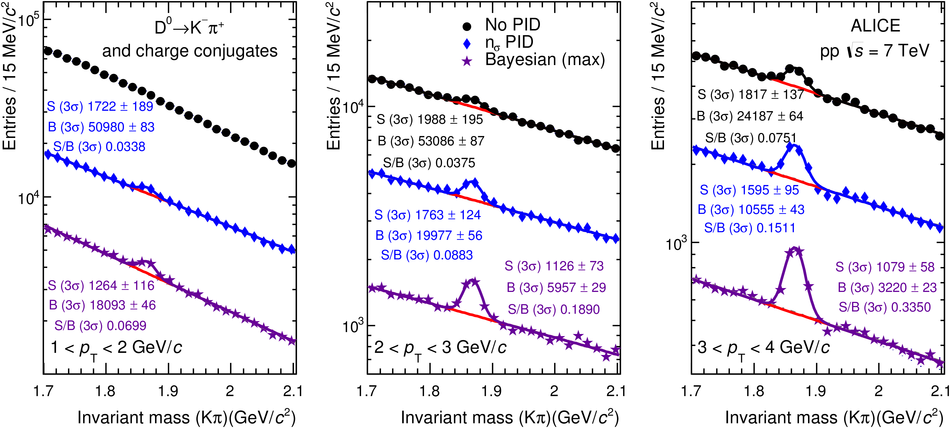## Figure 12

 (Left) Signal-to-background ratio and (right) statistical significance as a function of $\pt$ for various methods of particle identification. Note that the increase in significance at $8 < \pt < 12\,\gevc$ is an effect of the width of the $\pt$ interval increasing from 1 to 4 $\gevc$.## Figure 13

 A comparison of the PID efficiencies for $\Dzero$$\to$ K$^{-}\pi^{+}$ obtained using various PID strategies, as a function of $\pt$.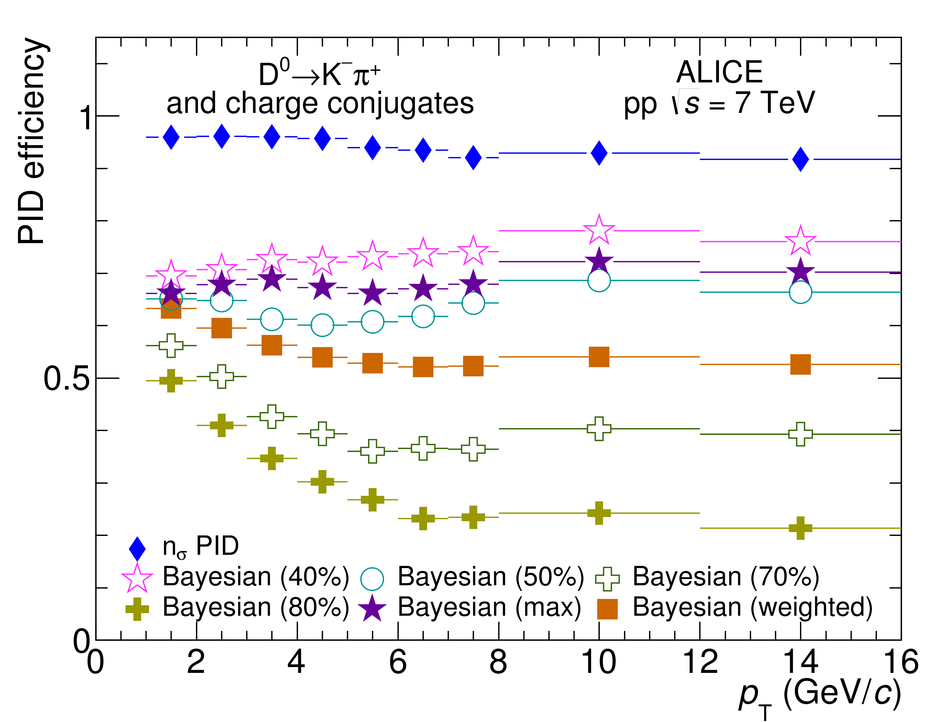## Figure 14

 Ratios of corrected yields obtained using various Bayesian PID methods to that obtained using n$_{\sigma}$ PID, for (left) fixed probability thresholds, and (right) maximum probability and weighted Bayesian PID, and no PID. The 5$\%$ systematic uncertainty on the n${_\sigma}$ PID method is shown as a blue box at 0.5 $\gevc$.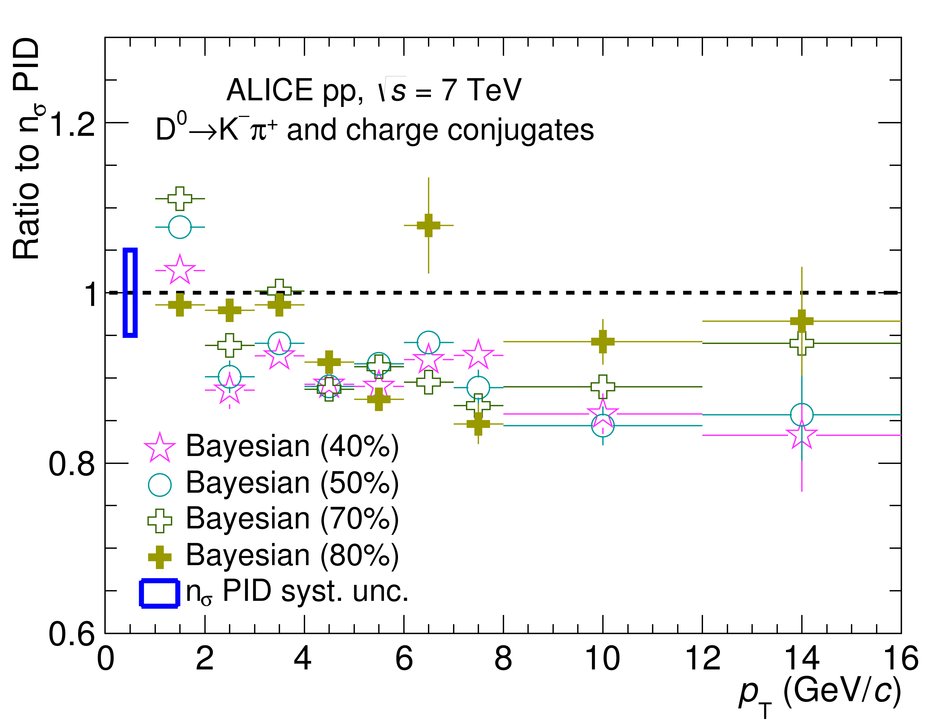## Figure 15

 Invariant mass spectra of $\Lambda^{+}_{c}\to$pK$^{-}\pi^{+}$ using n$_{\sigma}$ PID, minimum-$\sigma$ PID and Bayesian PID for (left) $2< \pt< 6$ GeV/$c$ and (right) $3< \pt< 4$ GeV/$c$. Due to the low statistical significance, it was not possible to extract a stable signal for n$_{\sigma}$ PID for $3< \pt< 4$ GeV/$c$, therefore this fit and its results are not shown.